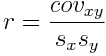The Pearson product-moment correlation coefficient is a numerical measure of the strength of a linear relationship between two numerical variables. Also called Pearson's r, it always has a value between -1 and +1. Values close to zero indicate little or no (linear) relationship between the two variables. Values far from zero indicate strong linear relationships.The formula is easy to remember if you remember that correlation is basically a rescaled covariance. The covariance is "like a variance," and the product of two standard deviations is "like a variance," so the correlation is "like a variance divided by a variance." In this case, it is how much the two variables vary together (the covariance) divided by how much the they vary separately (the product of the two standard deviations). Thus, the correlation coefficient is a "pure number." It has no units of measurement attached to it. Return To Main Page